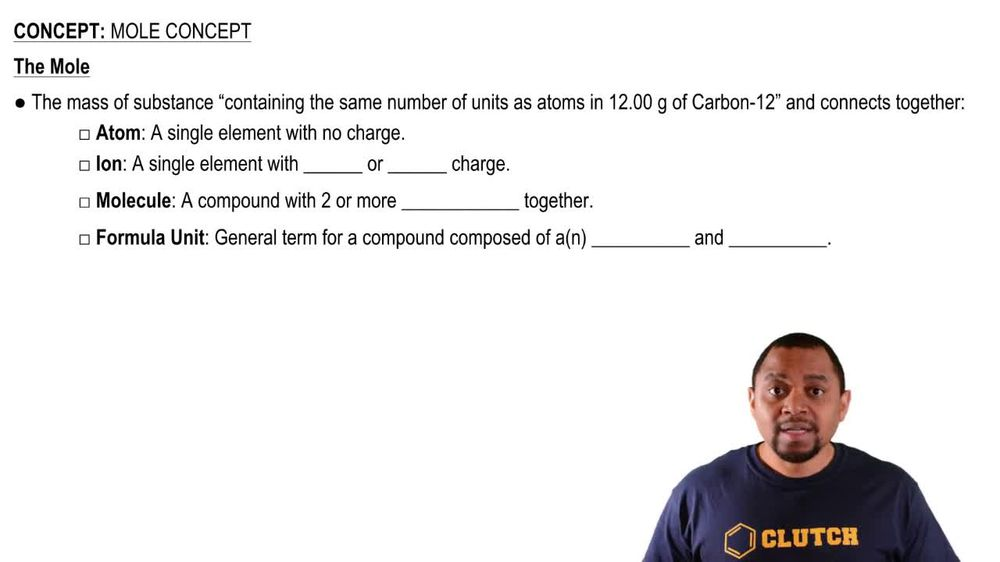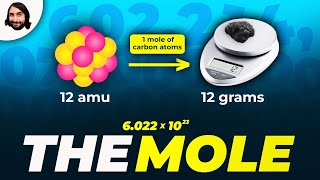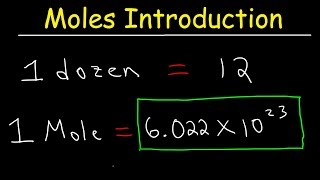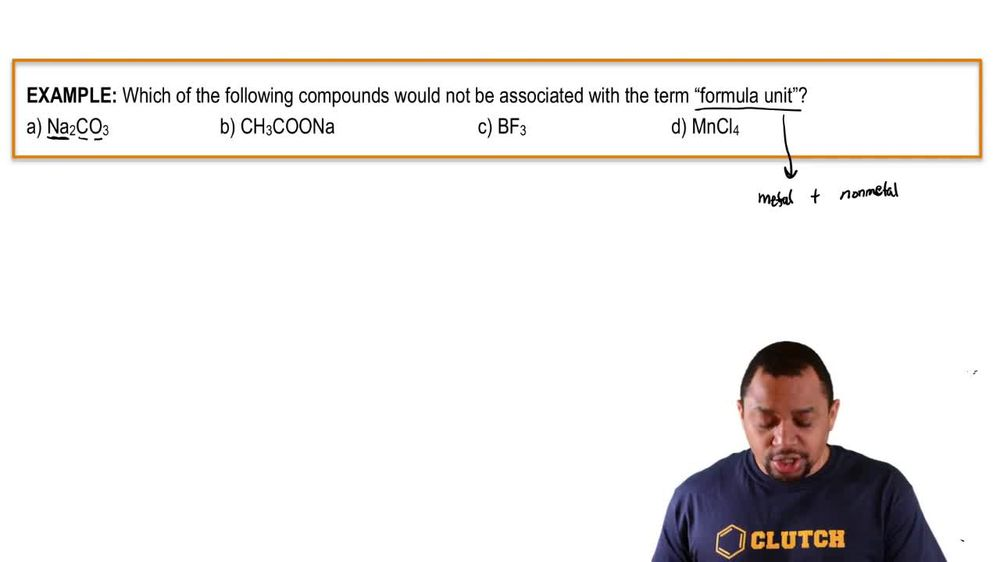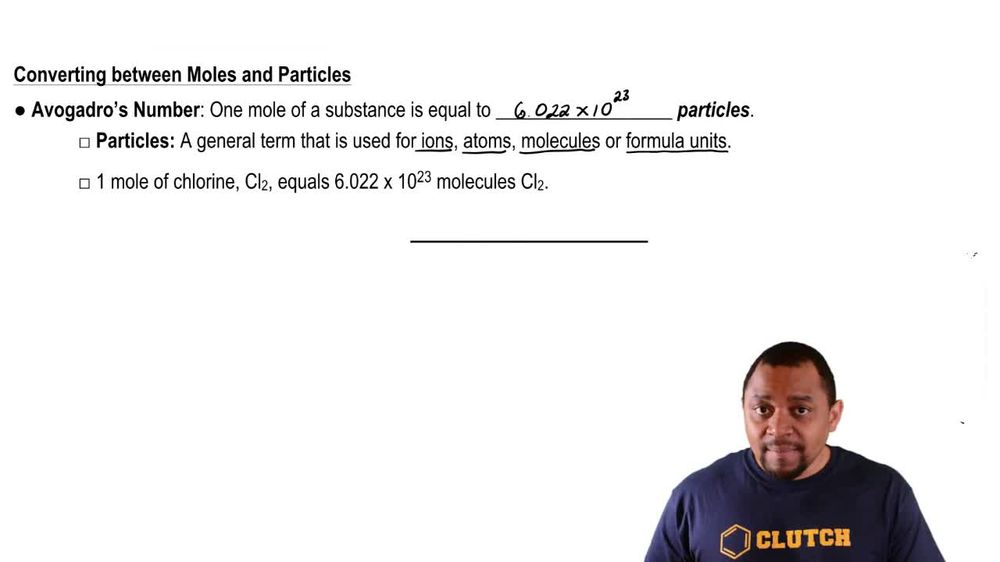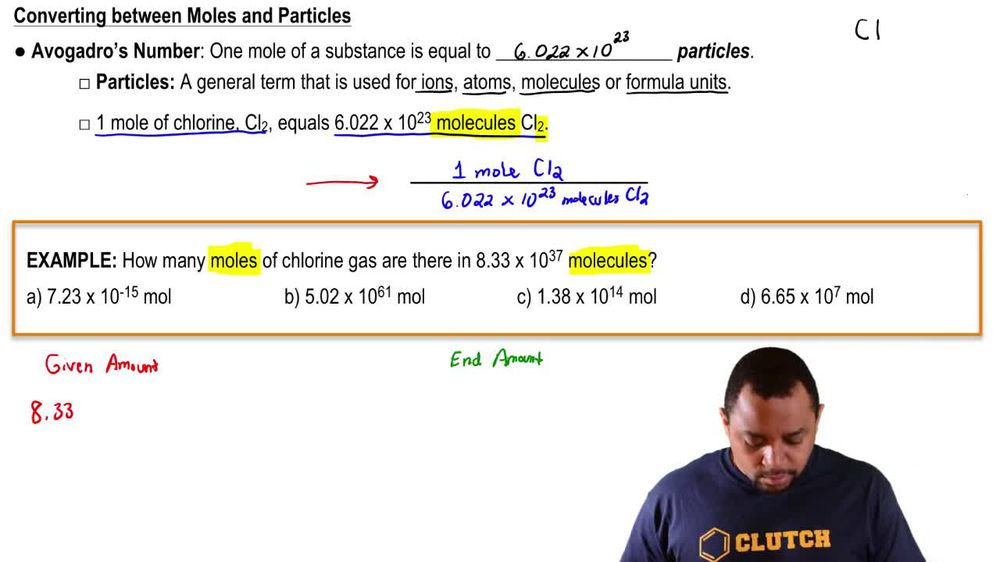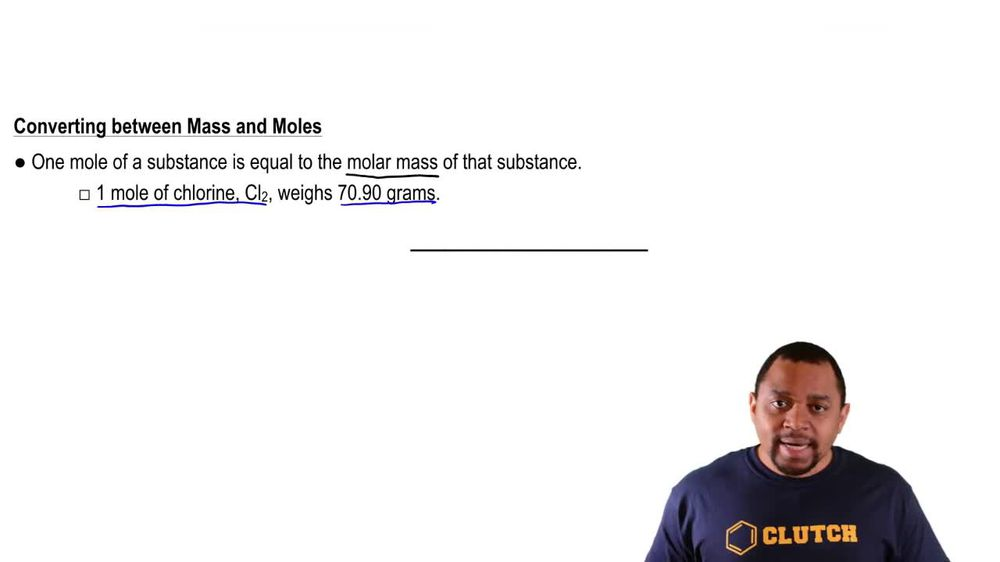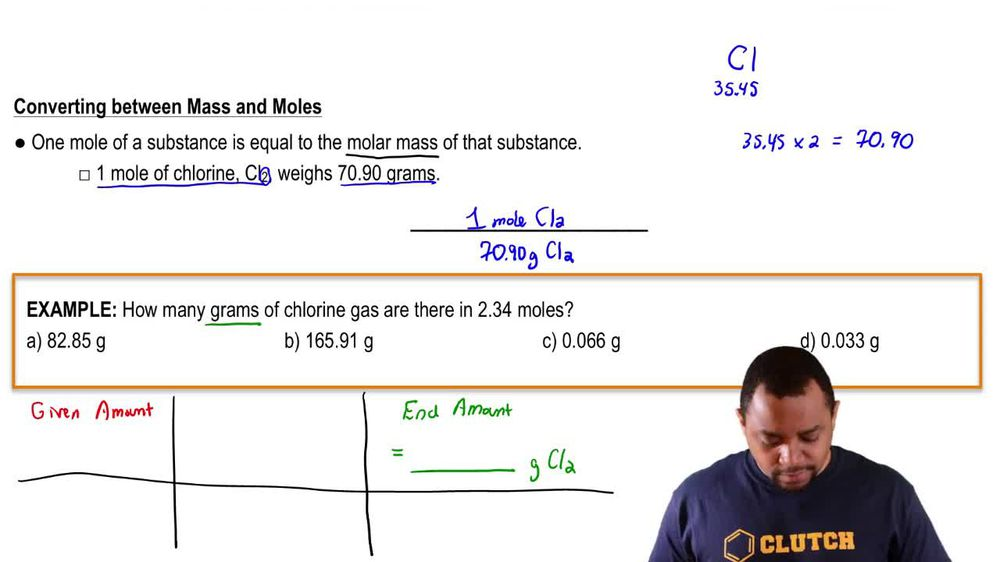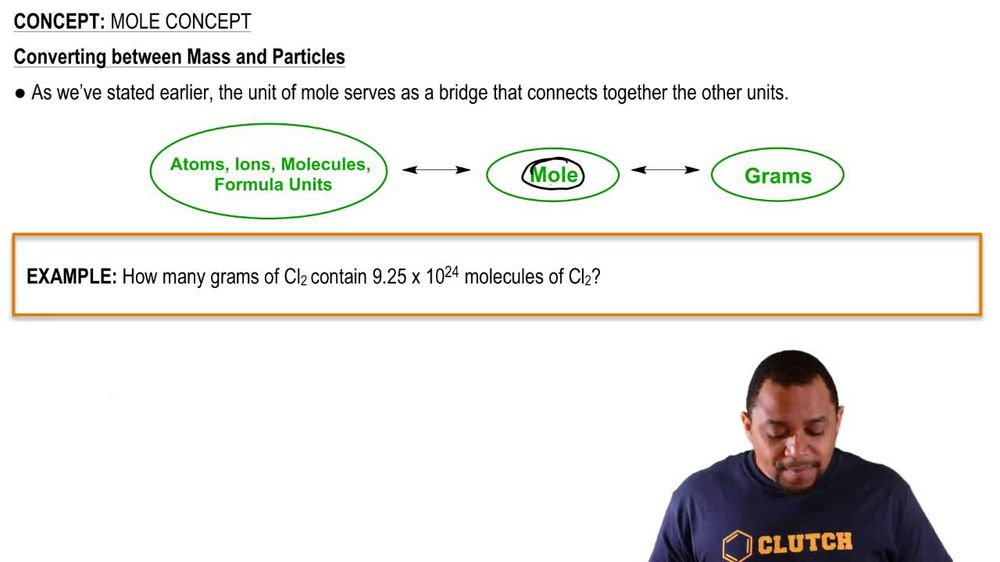Start typing, then use the up and down arrows to select an option from the list.
1. 2. Atoms & Elements2. Mole Concept
Problem

# Determine the number of moles (of molecules or formula units) in each sample. b. 23.6 kg Fe(NO3)2

Relevant Solution1m
Play a video:
Hey everyone. Our questionnaire asks us how many moles are in 175 kg of barium sulfide. We're first going to need to calculate our molar mass in order to answer this question So we can see that we have one of barium and we're going to multiply that by its atomic mass of 137.327 g per mole. And that's going to get us to 137.3 - seven. Next we have one of sulfur And we're going to multiply that by its atomic mass of 32.066, Which again will get us at 32.066 Adding these two up, we end up with a molar mass of 169. grams per mole. Now moving on to our question, we're going to start off with our 175 kg of barium sulfide And we want to convert this into Graham's first and we know that per one kg we have 10 to the 3rd g. And we're going to input our molar mass that we calculated and we know that we have 100 and 69.393 g per one mole of barium sulfide. And when we calculate this out and cancel out our units, we end up with a value of 1.03 times 10 to the third mole of barium sulfide, which is our final answer. So I hope this made sense. And let us know if you have any questions